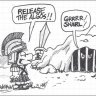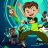# Can we get this supply and demand indicator?

W

#### whats up

##### Member
VIP
can we get this supply and demand indicator? ,like the one in the video i just posted thanks#### BenTen

Staff
VIP
Supply and Demand Zones are nothing but Support and Resistance (as noted by the YouTuber). It's better if you learn how to draw the S/R levels manually. An indicator won't do it justice for the stock. You can check out this indicator below.

Code:
``````# Mobius
# Support / Resistance
# V01.06.2012 V02.08.2013
# Added lower bar for clouds, Volume condition for plot and Vertical line for time.

input n = 13;
input ShowLines = yes;
input PlotTime = 1215; #hint PlotTime: Enter Bars End to plot Bars Start 0 for none.

def h = high;
def l = low;
def v = volume;
def Firstbar = barNumber();
def Highest = fold i = 1 to n + 1
with p = 1
while p
do h > getValue(h,-i);

def HVn = if V == Highest(v, n)
then l
else Double.NaN;

def A = if (Firstbar > n
and h == highest(h, n)
and Highest)
and HVn
then h
else double.NaN;
def Alow = if (Firstbar > n
and h == highest(h, n)
and Highest)
and HVn
then l
else double.nan;
def Lowest = fold j = 1 to n + 1
with q = 1
while q
do l < getValue(l, -j);
def B = if (Firstbar > n
and l == lowest(l, n)
and Lowest)
and HVn
then l
else double.NaN;
def Bhigh = if (Firstbar > n
and l == lowest(l, n)
and Lowest)
and HVn
then h
else double.nan;
def Al = if !isNaN(A)
then A
else Al;
def A2 = if !isNaN(Alow)
then Alow
else A2;
def Bl = if !isNaN(B)
then B
else Bl;
def B2 = if !isNaN(Bhigh)
then Bhigh
else B2;

plot ph = Round(A, 2);
ph.setPaintingStrategy(PaintingStrategy.VALUES_ABOVE);

plot hL = if Al > 0
then Al
else double.NaN;
hL.setHiding(!showLines);
hL.SetPaintingStrategy(PaintingStrategy.Dashes);
hL.SetDefaultColor(Color.Yellow);
plot hL2 = if A2 > 0
then A2
else double.nan;
hL2.setHiding(!showLines);
hL2.SetPaintingStrategy(PaintingStrategy.Dashes);
hL2.SetDefaultColor(Color.Yellow);

plot pl = Round(B, 2);
pl.setPaintingStrategy(PaintingStrategy.VALUES_BELOW);

plot ll = if Bl > 0
then Bl
else double.NaN;
ll.setHiding(!showLines);
ll.SetPaintingStrategy(PaintingStrategy.Dashes);
ll.SetDefaultColor(Color.Blue);
plot lH = if B2 > 0
then B2
else Double.NaN;
lH.setHiding(!showLines);
lH.SetPaintingStrategy(PaintingStrategy.Dashes);
lH.SetDefaultColor(Color.Blue);

# Time Markers
AddVerticalLine(SecondsTillTime(PlotTime) == 0, "", Color.Red, Curve.Short_Dash);

plot priceLine = highestAll(if isNaN(close[-1])
and !isNAN(close)
then close
else Double.NaN);
priceLine.SetStyle(Curve.Long_Dash);
priceLine.SetDefaultColor(CreateColor(75,75,75));
priceLine.SetLineWeight(1);
# End Code``````

•whats up and markos#### markos

##### Well-known member
VIP
There are several studies like this on the site. Type the word Pivot in the search box above and see what you come up with.

•whats up and BenTen#### markos

##### Well-known member
VIP
Or you could use fractal boxes...Last edited:
W

#### whats up

##### Member
VIP
Supply and Demand Zones are nothing but Support and Resistance (as noted by the YouTuber). It's better if you learn how to draw the S/R levels manually. An indicator won't do it justice for the stock. You can check out this indicator below.

Code:
``````# Mobius
# Support / Resistance
# V01.06.2012 V02.08.2013
# Added lower bar for clouds, Volume condition for plot and Vertical line for time.

input n = 13;
input ShowLines = yes;
input PlotTime = 1215; #hint PlotTime: Enter Bars End to plot Bars Start 0 for none.

def h = high;
def l = low;
def v = volume;
def Firstbar = barNumber();
def Highest = fold i = 1 to n + 1
with p = 1
while p
do h > getValue(h,-i);

def HVn = if V == Highest(v, n)
then l
else Double.NaN;

def A = if (Firstbar > n
and h == highest(h, n)
and Highest)
and HVn
then h
else double.NaN;
def Alow = if (Firstbar > n
and h == highest(h, n)
and Highest)
and HVn
then l
else double.nan;
def Lowest = fold j = 1 to n + 1
with q = 1
while q
do l < getValue(l, -j);
def B = if (Firstbar > n
and l == lowest(l, n)
and Lowest)
and HVn
then l
else double.NaN;
def Bhigh = if (Firstbar > n
and l == lowest(l, n)
and Lowest)
and HVn
then h
else double.nan;
def Al = if !isNaN(A)
then A
else Al;
def A2 = if !isNaN(Alow)
then Alow
else A2;
def Bl = if !isNaN(B)
then B
else Bl;
def B2 = if !isNaN(Bhigh)
then Bhigh
else B2;

plot ph = Round(A, 2);
ph.setPaintingStrategy(PaintingStrategy.VALUES_ABOVE);

plot hL = if Al > 0
then Al
else double.NaN;
hL.setHiding(!showLines);
hL.SetPaintingStrategy(PaintingStrategy.Dashes);
hL.SetDefaultColor(Color.Yellow);
plot hL2 = if A2 > 0
then A2
else double.nan;
hL2.setHiding(!showLines);
hL2.SetPaintingStrategy(PaintingStrategy.Dashes);
hL2.SetDefaultColor(Color.Yellow);

plot pl = Round(B, 2);
pl.setPaintingStrategy(PaintingStrategy.VALUES_BELOW);

plot ll = if Bl > 0
then Bl
else double.NaN;
ll.setHiding(!showLines);
ll.SetPaintingStrategy(PaintingStrategy.Dashes);
ll.SetDefaultColor(Color.Blue);
plot lH = if B2 > 0
then B2
else Double.NaN;
lH.setHiding(!showLines);
lH.SetPaintingStrategy(PaintingStrategy.Dashes);
lH.SetDefaultColor(Color.Blue);

# Time Markers
AddVerticalLine(SecondsTillTime(PlotTime) == 0, "", Color.Red, Curve.Short_Dash);

plot priceLine = highestAll(if isNaN(close[-1])
and !isNAN(close)
then close
else Double.NaN);
priceLine.SetStyle(Curve.Long_Dash);
priceLine.SetDefaultColor(CreateColor(75,75,75));
priceLine.SetLineWeight(1);
# End Code``````
thank you so much for your response
I will try it

Last edited by a moderator:
•markos
J

#### john3

##### Active member
2019 Donor
@markos What are fractal boxes?#### markos

##### Well-known member
VIP
@markos What are fractal boxes?
@john3 I put it in a separate thread on this site. They are basically areas of support/resistance in a given time frame. You can then see when it escapes the box. Markets are fractal, but I have no idea how this would work or be of benefit on small aggs/ timeframes. Hope that helps.

•whats up
R

#### roger80k

##### New member
Hi Guys,

Can you please help me locate this type of MTF Supply Zone Script. As shown in this Youtube Video that will show the supply zone with the time frame placed on the box.

Thanks#### BenTen

Staff
VIP
@roger80k If I'm not mistaken, that was drawn manually.

R

#### roger80k

##### New member
@roger80k If I'm not mistaken, that was drawn manually.

Ben is There a way to add 2 different time frames into the supply and demand zones. For example: Display the 4 hour demand and daily demand without having to run multiple charts. Thanks#### BenTen

Staff
VIP
@roger80k I'm not getting your question. Are you referring to a specific indicator?

B

#### Beltrame1

##### New member
VIP
Need help fixing it this supply and demand zones indicator.

Code:
``````//AUTO : DEMAND AND SUPPLY ZONE
//Date : 18th Aug 2019

_SECTION_BEGIN("Demand");
SetChartOptions(0,chartShowArrows|chartShowDates);
bk=ParamColor( "Bk col",ColorRGB(85,90,60));
SetChartBkColor(bk);

amount = Param("Sensitivity", 0.5, 0.1, 2, 0.1 );

array = C ;
zz0 = Zig( array, amount );
zz1 = Ref( zz0, -1 );
zz2 = Ref( zz0, -2 );

tr = ValueWhen(zz0 > zz1 AND zz1 < zz2, zz1);
pk = ValueWhen(zz0 < zz1 AND zz1 > zz2, zz1);
PU = tr + 0.01 * abs(tr)*amount;
PD = pk - 0.01 * abs(pk)*amount;

ZZT = IIf( array >= PU AND zz0 > zz1, 1,
IIf( array <= PD AND zz0 < zz1, -1, 0 ) );

ZZT = ValueWhen( ZZT != 0, ZZT );

Sell_Valid_=zzt<0;
Plot(Ref(Sell_valid,0),"",ColorRGB(100,0,0),styleHistogram|styleDashed|styleOwnScale|styleNoLabel,0,0,0,-1);

SetBarFillColor(Cc1);
Plot(C,"Demand & Supply Zone AFL By NTA Blogger",Cc1,64,0,0,0,0);

Ll=LowestSince(sell_valid,L,1);
Llm=LowestSince(sell_valid,Min(O,C),1);

xx=Cum(1);
NoLines = Param("No of Lines",5,1,10,1);

Col2=ParamColor( "Res Color", colorRed );
Col1=ParamColor( "Sup Color", colorGreen );

for( i = 1; i < NoLines+1 ; i++ )
{
scol=ColorBlend(Col1,2,0.1*i);
rcol=ColorBlend(Col2,2,0.1*i);

pz1 = LineArray(px1, py1, (BarCount-1), py1);
Plot(pz1,"",scol,32);

tx1 = LastValue(ValueWhen(sell_valid,Cum(1),i)) ;
ty1 = LastValue(ValueWhen(sell_valid,Hh,i)) ;
tz1 = LineArray(tx1, ty1, (BarCount-1), ty1);
Plot(tz1,"",rcol,32);

pz1m = LineArray(px1m, py1m, (BarCount-1), py1m);
PlotOHLC(pz1,pz1,pz1m,pz1m,"",scol,styleCloud|styleNoLabel,0,0,0,-i-nolines);

tx1m = LastValue(ValueWhen(sell_valid,Cum(1),i)) ;
ty1m = LastValue(ValueWhen(sell_valid,Hhm,i)) ;
tz1m = LineArray(tx1m, ty1m, (BarCount-1), ty1m);
PlotOHLC(tz1,tz1,tz1m,tz1m,"",rcol,styleCloud|styleNoLabel,0,0,0,-i-nolines);
}

for( j = 0; j < BarCount; j++ )
{
if( Buy_valid [j])  PlotText( "Dz\n"+Ll[ j ], j, Ll[j], colorPaleGreen );
if( Sell_valid[j] ) PlotText( "Sz\n"+Hh[ j ], j, Hh[j], colorRose);
}
_SECTION_END();``````

Last edited by a moderator:#### BenTen

Staff
VIP
@Beltrame1 That's not even thinkscript code.

•Bobbydigital83
B

#### Beltrame1

##### New member
VIP
@BenTen Sorry, I didn`t know the difference... LOL. Thanks

T

#### Ty199

##### New member
There is a good supply and demand indicator for NT. How do you get it over to TOS ? I tried to open the download but I have no app that will open it to read it .

A

#### Alex151

##### New member
There is a good supply and demand indicator for NT. How do you get it over to TOS ? I tried to open the download but I have no app that will open it to read it .
Which one? I use TOS for charting and NT8 for execution.

T

#### Ty199

##### New member
Which one? I use TOS for charting and NT8 for execution.
I cant find it. Its on my NT. It was free from somewhere. How can you pull a file from NT

T

#### Ty199

##### New member
@Alex151 I tried to export it but it would only do a zip file. I cant load it on here. But its free under Indicator warehouse.comFresh Supply & Demand Levels for ThinkorSwim Custom 0Supply and Demand Candles for ThinkorSwim Custom 0ZigZag High Low with Supply & Demand Zones for ThinkorSwim Indicators 44